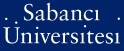# Factorization of a class of polynomials over finite fields

Stichtenoth, Henning and Topuzoğlu, Alev (2012) Factorization of a class of polynomials over finite fields. Finite Fields and Their Applications, 18 (1). pp. 108-122. ISSN 1071-5797

This is the latest version of this item.

Full text not available from this repository.

Official URL: http://dx.doi.org/10.1016/j.ffa.2011.07.005

## Abstract

We study the factorization of polynomials of the form F(r)(X) = bx(qr+1) - ax(qr) + dx - c over the finite field F(q). We show that these polynomials are closely related to a natural action of the projective linear group PGL(2, q) on non-linear irreducible polynomials over F(q). Namely, irreducible factors of F(r)(X) are exactly those polynomials that are invariant under the action of some non-trivial element [A] is an element of PGL(2, q). This connection enables us to enumerate irreducibles which are invariant under [A]. Since the class of polynomials F(r)(x) includes some interesting polynomials like x(qr) - x or x(qr+1) - 1, our work generalizes well-known asymptotic results about the number of irreducible polynomials and the number of self-reciprocal irreducible polynomials over F(q). At the same time, we generalize recent results about certain invariant polynomials over the binary field F(2).

Item Type: Article Polynomial factorization; Self-reciprocal polynomial; Group action on irreducible polynomials; Invariant polynomial Q Science > QA Mathematics 18941 Henning Stichtenoth 29 Mar 2012 12:07 31 Jul 2019 10:27

### Available Versions of this Item

Repository Staff Only: item control page